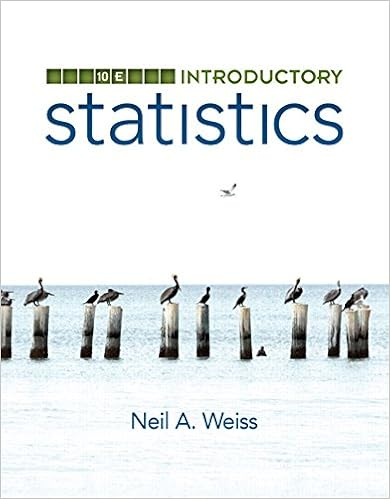# A use dynamic geometry software to construct a right

• 66

This preview shows page 12 - 15 out of 66 pages.

a.Use dynamic geometry software to construct a right triangle with acute anglemeasures of 30°and 60°(a 30°- 60°- 90°triangle), where the shorter leg lengthis 3 units.b.Find the exact ratiosof the side lengths(using square roots).ABAC=0321451043215ACBABBC=ACBC=c.Repeat parts (a) and (b) for several other 30°- 60°- 90°triangles. Use your resultsto write a conjecture about the ratios of the side lengths of a 30°- 60°- 90°triangle.Communicate Your AnswerCommunicate Your Answer3.What is the relationship among the side lengths of 45°- 45°- 90°triangles?30°- 60°- 90°triangles?USING PRECISEMATHEMATICALLANGUAGETo be proficient in math,you need to expressnumerical answers witha degree of precisionappropriate for theproblem context.SamplePointsA(0, 4)B(4, 0)C(0, 0)SegmentsAB=5.66BC=4AC=4AnglesmA=45°mB=45°SamplePointsA(0, 5.20)B(3, 0)C(0, 0)SegmentsAB=6BC=3AC=5.20AnglesmA=30°mB=60°G.9.BTEXASESSENTIALKNOWLEDGEANDSKILLS
##### We have textbook solutions for you!The document you are viewing contains questions related to this textbook.
Chapter A / Exercise 32
Precalculus: Mathematics for Calculus
Redlin/StewartExpert VerifiedBrowse all Textbook Solutions
9.2LessonWhat You Will LearnWhat You Will LearnFind side lengths in special right triangles.Solve real-life problems involving special right triangles.Finding Side Lengths in Special Right TrianglesA 45°- 45°- 90°triangle is anisosceles right trianglethat can be formed by cutting asquare in half diagonally.Finding Side Lengths in 45°- 45°- 90°TrianglesFind the value ofx. Write your answer in simplest form.a.x845°b.xx52SOLUTION2.REMEMBERA radical with index 2is in simplest form whenno radicands have perfectsquares as factors otherthan 1, no radicandscontain fractions, andno radicals appear in thedenominator of a fraction.Previousisosceles triangleCore VocabularyCore VocabularyTheoremTheoremTheorem 9.445°- 45°- 90°Triangle TheoremIn a 45°- 45°- 90°triangle, the hypotenuse is2 times as long as each leg.ProofEx. 19, p. 480hypotenuse=leg2xxx245°45°
b.By the Base Angles Theorem (Theorem 5.6) and the Corollary to the Triangle SumTheorem (Corollary 5.1), the triangle is a 45°- 45°- 90°triangle.
476Chapter 9Right Triangles and Trigonometry
Section 9.2Special Right Triangles477Finding Side Lengths in a 30°- 60°- 90°TriangleFind the values ofxandy. Write your answerin simplest form.SOLUTIONStep 1Find the value ofx.

Course Hero member to access this document

Course Hero member to access this document

End of preview. Want to read all 66 pages?

Course Hero member to access this document

Term
Fall
Professor
Michael McCall
Tags
Right triangle, triangle, Triangle Theorem
##### We have textbook solutions for you!
The document you are viewing contains questions related to this textbook.The document you are viewing contains questions related to this textbook.
Chapter A / Exercise 32
Precalculus: Mathematics for Calculus
Redlin/StewartExpert Verified
•••